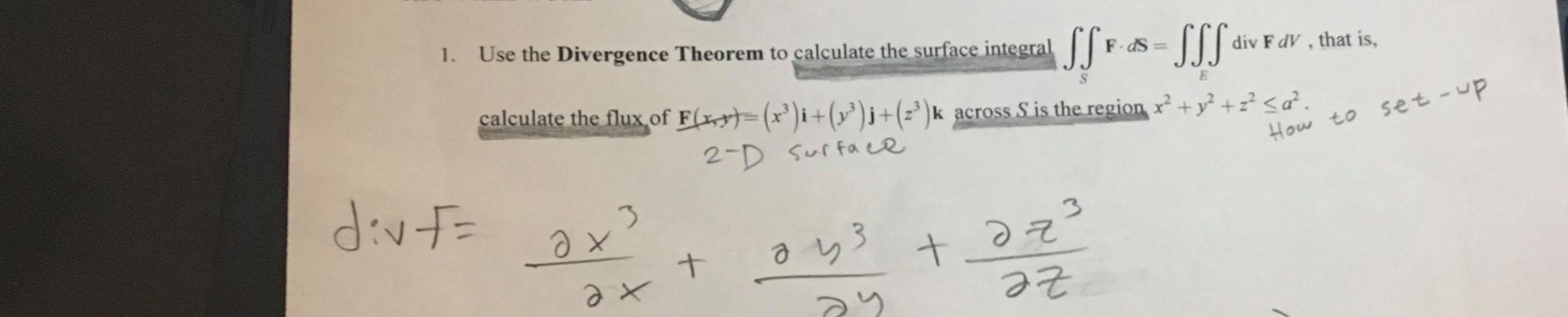# Use the Divergence Theorem to calculate the surface integral F dS =|1.-SSSdiv F dV , that is,calculate the flux of F(x=(x')i+(y³)j+(z*)k across S is the region x +y² +z?

Question
2 views

How do i set up the problem? if some can explain the set up i would very much appreciate ithelp_outlineImage TranscriptioncloseUse the Divergence Theorem to calculate the surface integral F dS =| 1. -SSS div F dV , that is, calculate the flux of F(x=(x')i+(y³)j+(z*)k across S is the region x +y² +z?
check_circle

Step 1

Given,

Step 2

Divergence theorem states that

Step 3

The divergence ...

### Want to see the full answer?

See Solution

#### Want to see this answer and more?

Solutions are written by subject experts who are available 24/7. Questions are typically answered within 1 hour.*

See Solution
*Response times may vary by subject and question.
Tagged in

### Integration